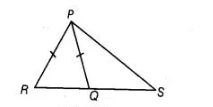# If Q is a point oh the side SR of a ΔPSR`
Question:

If Q is a point oh the side SR of a ΔPSR such that PQ = PR, then prove that PS > PQ.

Thinking Process

Use the property of a triangle that if two sides are equal then their opposite angles are also equal. Also use the property that side opposite to greater

angle is longer.

Solution:

Given in $\triangle P S R, Q$ is a point on the side $S R$ such that $P Q=P R$.

To prove $P S>P Q$Proof $\ln \Delta P R Q$, $P Q=P R$ [given]

$\Rightarrow$ $\angle R=\angle P Q R$ ...(i)

[angles opposite to equal sides are equal]

But $\angle P Q R>\angle S$ ...(ii)

[exterior angle of a triangle is greater than each of the opposite interior angle]

From Eqs. (i) and (ii), $\angle R>\angle S$

$\Rightarrow \quad P S>P R$ [side opposite to greater angle is longer]

$\Rightarrow$ $P S>P Q$ $[\because P Q=P R]$